# Full Wave Rectifier

## Full wave Rectifier

In full wave rectifier , current flows through the load in the same direction ( i.e. d.c.) for both the half cycles of input a.c. supply voltage. There are two types of circuits commonly used for full-wave rectifications:

• Centre-tap full wave rectifier
• Full wave bridge rectifier

### Centre-tap  Full wave Rectifier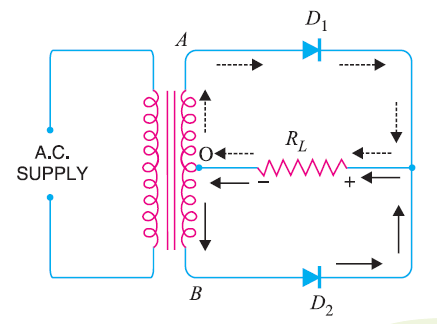Fig.1

Fig.2

Fig(1) shows the circuit diagram of a Centre-tap full wave rectifier and  Fig(2) shows the input and output waveform of a centre-tap full wave rectifier .

The circuits uses two diodes D1 and D2. A centre-tap secondary winding AB is connected with the two diodes such that each diode uses one half-cycle of input a.c. voltage. That means diode Dutilises the the upper half of secondary winding for rectification and diode D2  uses the lower half.

Operation:

During the positive half cycle of secondary voltage, the end A of the secondary winding  becomes positive and end B negative.

So diode  D1 is forward biased and diode D2 is reverse biased. Hence, diode D1 conducts and diode D2 does not.

The current flows through diode D1, load resistance RL and the upper half of the secondary winding OA. This is shown by the dotted arrows.

During the negative half cycle of secondary voltage, the end A of the secondary winding  becomes negative and end B positive.

So diode D2 is forward biased and  D1 is reverse biased. Hence D2 conducts while D1 does not.

The conventional current flows through diode D2 , load resistance RL and the lower half of thr secondary winding OB as shown by the solid arrows.

As we can see that current in the load RL  flows in the same direction for both the half cycles of input supply voltage. So d.c. is  obtained across RL.

Peak Inverse Voltage:

Let Vm is the maximum voltage across the half secondary winding. Fig() shows the circuit at the instant secondary voltage reaches its maximum value Vm in the positive direction.At this instant diode D1 is conducting and D2is not conducting.So whole of the secondary voltage appears across the non-conducting diode.Hence the peak inverse voltage is twice the maximum voltage across the half secondary winding.

PIV = 2 Vm

It is difficult to locate the centre tap on secondary winding.

The d.c. output is low as each diode utilizes only half of the secondary voltage.

The diodes must have high peak inverse voltage.

### Full Wave Bridge Rectifier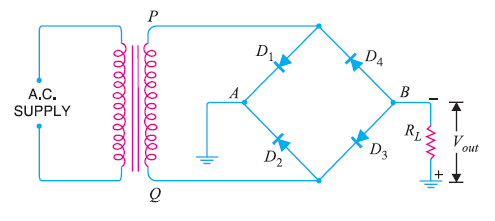Fig. 3

Fig(3) shows the circuit connection of a full wave bridge rectifier and Fig(6) shows the input and output waveform of  full-wave bridge rectifier.

The full wave  bridge rectifier circuit  contains four diodes D1 , D2,D3 and D4, connected to form a bridge as shown in Fig(4).

The a.c. supply to be rectified is applied to the diagonally opposite ends of the bridge through the transformer. Between other two ends of the bridge, the load resistance RL is connected.

Operation:

During the positive half-cycle of secondary voltage, the end P of the secondary winding becomes positive and end Q negative.

This makes diodes D1 and Dforward biased while Dand D4 are reverse biased. Hence  only diodes  D1 and D3 conducts.

These two diodes will be in series through the load RL as shown in Fig. 5 .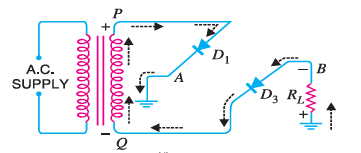Fig.5

The conventional current flows through load RL is shown by the dotted arrows. It may be seen that current flows from A to B through the load RL .

During the negative half cycle of secondary voltage, end P becomes negative and end Q positive.

This makes diodes D2 and D4and forward biased  and  diodes D1 and Dare reverse biased. Hence only diodes Dand D4  conducts.

These two diodes will be in series through the load RL as shown in Fig. 6 .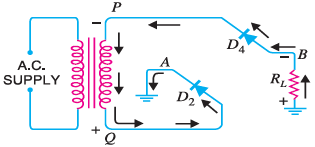Fig.6

The conventional current flow through load Ris shown by the solid arrows.

It may be seen that again the current flows from A to B through the load i.e. in the same direction as for the positive half-cycle.
Therefore, d.c. output is obtained across load RL.

### Peak Inverse Voltage

The peak inverse voltage(PIV) of each diode is equal to the maximum secondary voltage of transformer  i.e. Vm.

Suppose during positive half cycle of input a.c., end P of secondary is positive and end Q negative. Under such conditions, diodes D1 and D3 are forward biased while diodes D2 and D4 are reverse biased. Since the diodes are considered ideal, diodes D1 and D3 can be replaced by wires as shown in Fig. 7 (i). This circuit is the same as shown in Fig. 7 (ii).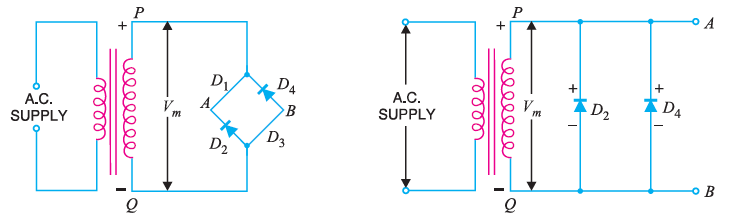(i)                                                                                                      (ii)

Fig.7

From the Fig. 7 (ii), it is clear that two reverse biased diodes (i.e., D2 and D4) and the secondary of transformer are in parallel. Hence PIV of each diode (D2 and D4) is equal to the maximum voltage (Vm) across the secondary.

Similarly, during the next half cycle, D2 and D4 are forward biased while D1 and D3 will be reverse biased. It is easy to see that reverse voltage across D1 and D3 is equal to Vm.

Hence, PIV  =  Vm

1. There is no need of centre taped transformer in full-wave bridge rectifier.
2. The output is twice that of centre-tap circuit for the same secondary voltage.
3. The PIV is half that of the centre-tap circuit.

1. It needs four diodes.

Since during each half cycle two diodes that conducts are in series so voltage drop in the internal resistance of the rectifying unit is twice as in the centre-tap circuit. This is objectionable when secondary voltage is small.

### Output Frequency of Full Wave Rectifier

The output frequency of a full wave rectifier is double the input frequency.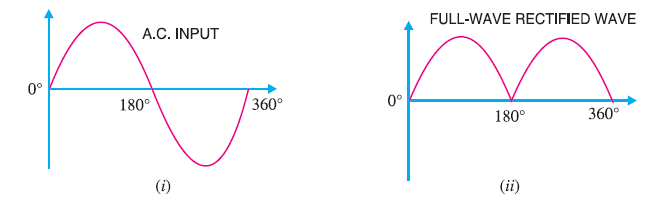Fig. 8

As we know, a wave has a complete cycle when it repeats the same pattern. In Fig. 8 (i), the input a.c. completes one
cycle from 0° – 360°. However, the full-wave rectified wave completes 2 cycles in this period As shown in Fig.8(ii). Therefore, output frequency is twice the input frequency i.e.

fout= 2fin

### Efficiency of Full Wave Rectifier

Fig.9 shows the full wave rectification process.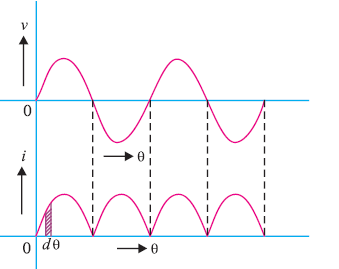Fig.9

Let  v = Vmsinθ (the a.c. voltage to be rectified)

rf = diode resistance

i= instantaneous currentEfficiency η: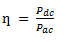#### d.c. output power

The output current is pulsating direct current. Therefore, in order to find the d.c. power, average current has to be found out.

Let  Idc=  Iav =average current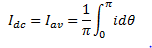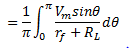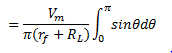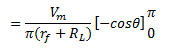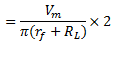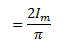So d.c.power output,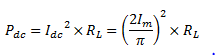#### a.c. input power

The a.c. input power is given by :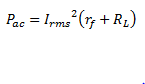For  a full-wave rectified wave,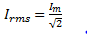Full-wave rectification efficiency η is :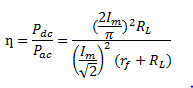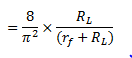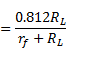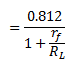The efficiency will be maximum if rf is negligible as compared to RL

So Maximum Efficiency  = 81.2%

This is double the efficiency of a half wave rectifier .Therefore,a full wave rectifier is twice as effective as a half-wave rectifier.

#### Ripple Factor

The output of a rectifier consists of a d.c. component and an a.c. component, which is also known as ripple.

The a.c. component is undesirable and accounts for the pulsations in the rectifier output.

The effectiveness of a rectifier depends upon the magnitude of a.c. component in the output. i.e., the smaller this
component, the more effective is the rectifier.

The ratio of r.m.s. value of a.c. component to the d.c. component in the rectifier output is known as ripple factor i.e.

Ripple factor = r.m.s. value of a.c component/value of d.c. component = Iac /Idc

In full-wave rectification,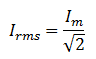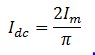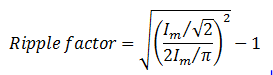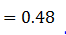It is clear that d.c. component exceeds the a.c. component in the output of a full wave rectifier .This results in lesser pulsation in the output of a full wave rectifier as compared to a half wave rectifier . Therefore, full-wave rectification is invariably used for conversion rectification.